Exponentially faster implementations of Select(H) for
fermionic Hamiltonians
Kianna Wan
1,2
1
Stanford Institute for Theoretical Physics, Stanford University, Stanford, CA 94305, USA
2
PsiQuantum, Palo Alto, CA 94304, USA
We present a simple but general framework for constructing quantum circuits that im-
plement the multiply-controlled unitary Select(H)
:
=
P

|ih| H

, where H =
P

H

is the Jordan-Wigner transform of an arbitrary second-quantised fermionic Hamiltonian.
Select(H) is one of the main subroutines of several quantum algorithms, including state-
of-the-art techniques for Hamiltonian simulation. If each term in the second-quantised
Hamiltonian involves at most k spin-orbitals and k is a constant independent of the to-
tal number of spin-orbitals n (as is the case for the majority of quantum chemistry and
condensed matter models considered in the literature, for which k is typically 2 or 4), our
implementation of Select(H) requires no ancilla qubits and uses O(n) Cliﬀord+T gates,
with the Cliﬀord gates applied in O(log
2
n) layers and the T gates in O(log n) layers. This
achieves an exponential improvement in both Cliﬀord- and T -depth over previous work,
while maintaining linear gate count and reducing the number of ancillae to zero.
1 Introduction
Quantum computers have the potential to eﬃciently simulate quantum systems. A particularly promis-
ing application of both near-term and fault-tolerant architectures is solving problems in quantum
chemistry and materials science. In recent years, signiﬁcant advances have been made on this front;
for reviews of the major algorithmic developments, we refer the reader to Refs. .
Much of the current research in quantum simulation is concerned with the estimation of Hamiltonian
spectra and preparation of energy eigenstates, which can provide insight into various properties of
molecules and materials. As shown by Ref. , the quantum phase estimation algorithm [5, 6] can be
used to perform projective energy measurements, collapsing the system into a desired eigenstate with
high probability if the initial state has appreciable overlap with that eigenstate. Even in the absence
of a suitable initial approximation, such measurements may be applied to prepare an eigenstate by
exploiting the quantum Zeno eﬀect [7, 8]. Alternatively, approximate eigenstates may be obtained
via adiabatic state preparation, given suﬃcient information about the gap(s) in the spectrum of the
interpolating Hamiltonian [9, 10].
Several techniques are useful for realising these schemes on a gate-based quantum computer.
For instance, the qubitisation procedure of Refs. [11, 12] can implement the time-evolution opera-
tor exp(iHt) or, more directly, a walk operator corresponding to exp[i arccos(Ht)], for a time-
independent Hamiltonian H and some t R. Either of these operators can be used as the unitary input
to phase estimation for the purpose of approximating eigenvalues and eigenstates of H [4, 8, 13, 14].
Adiabatic evolution can be digitally simulated by applying the truncated Dyson series algorithm of
Refs. [15, 16] for time-dependent Hamiltonian simulation, or by using the method of Ref. , which is
based on quasi-adiabatic continuation . Approximate ground states can also be prepared using the
Kianna Wan: kianna@stanford.edu, This research was completed during an internship at PsiQuantum.
Accepted in Quantum 2021-01-04, click title to verify. Published under CC-BY 4.0. 1
arXiv:2004.04170v3 [quant-ph] 7 Jan 2021methods of Ref. [19, 20]. All of these techniques are formulated in terms of queries to unitary oracles
that encode the relevant Hamiltonian(s) in some form. One such encoding is the “linear combination
of unitaries” (LCU) query model, motivated by the algorithms of Refs. [21, 22]. In this model, the
input Hamiltonian is decomposed as
H =
L1
X
=0
α

H

,
where each H

is a time-independent unitary and the (possibly time-dependent) coeﬃcients α

are
real and nonnegative. Information about the Hamiltonian is accessed via two oracles: Select(H) and
Prepare(α), which respectively encode the unitaries H

and the coeﬃcients α

. Speciﬁcally,
Select(H)
:
=
L1
X
=0
|ih| H

(1)
is a multiply-controlled operation that applies the unitary H

to the target register conditioned on the
control register being in the state |i, and Prepare(α) is some unitary that transforms the all-zeros
state of the control register as
Prepare(α) : |0i 7→
L1
X
=0
r
α

α
|i,
where α
:
=
P
L1
=0
α

. (In the case where the coeﬃcients are time-dependent, Prepare(α) may be
controlled on an additional register that encodes time .)
While any operator can in principle be written as a linear combination of unitaries, some Hamil-
tonians are more naturally expressed in this framework. Since the complexities of the aforementioned
algorithms are typically dominated by that of Select(H) and Prepare(α),
1
it is important to de-
sign time- and space-eﬃcient circuits for these oracles. The purpose of this paper is to provide an
eﬃcient construction for Select(H) in the case where H is obtained from a fermionic Hamiltonian
via the Jordan-Wigner transformation , so that each H

is a tensor product of Pauli operators.
Although our method is applicable to arbitrary fermionic Hamiltonians, it is worth noting that in
many of the models considered in practice, each site interacts with only a small number of other sites.
More precisely, for a fermionic Hamiltonian given in its second-quantised representation, let k denote
the maximum number of distinct spin-orbitals that appear in each term. For most Hamiltonians of
physical interest, such as the commonly studied molecular electronic structure Hamiltonian and the
Fermi-Hubbard model, k does not scale with the system size. Our contribution can be stated as follows.
Main result: For any fermionic Hamiltonian for which k is a constant independent of the number of
spin-orbitals n, we can construct a circuit for Select(H) using zero ancilla qubits and O(n) Cliﬀord
and T gates, with the Cliﬀord gates performed in O(log
2
n) layers and the T gates in O(log n) layers.
This constitutes an exponential reduction in Cliﬀord- and T -depth compared to existing methods. The
approach of Ref.  can be applied to arbitrary LCU inputs but requires O(L) Cliﬀord and T gates
and O(L) Cliﬀord- and T -depth, and in general L O(n
k
) for the type of Hamiltonians considered
here. Ref.  improves the gate count and depth (for both Cliﬀord and T gates) to O(n) for two
speciﬁc k = 2 Hamiltonians. Like Ref. , we obtain a speedup by exploiting the structure of the
Jordan-Wigner encoding.
2
However, our circuits are completely diﬀerent in structure from those in
1
Strictly speaking, these algorithms make calls to controlled versions of Select(H) and Prepare(α). In our im-
plementation, a constant number of controls can be added to Select(H) with constant additive overhead in the gate
complexity, as will become clear in Section 2.
2
Although we focus on the Jordan-Wigner transformation in this paper, the same ideas can be used to eﬃciently
Accepted in Quantum 2021-01-04, click title to verify. Published under CC-BY 4.0. 2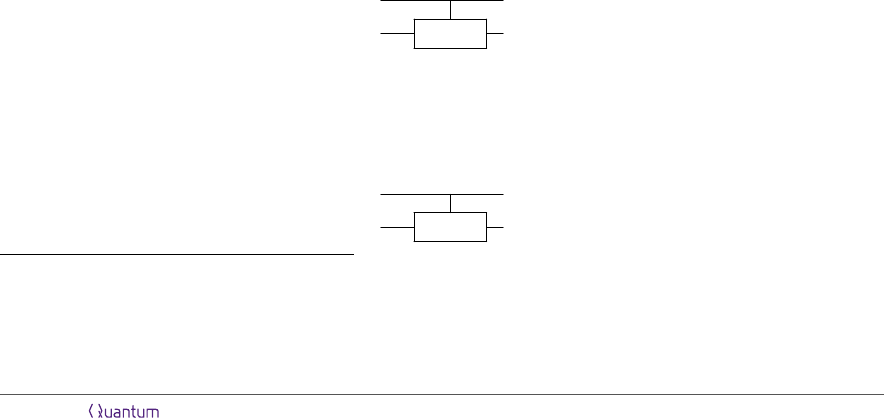Ref. , which cannot be parallelised to sublinear-depth in any straightforward way. Moreover, our
implementation uses no ancilla qubits, in contrast to the log n required by Refs.  and .
Our construction can be directly applied to asymptotically improve the circuit depth of existing
fermionic simulation algorithms that are bottlenecked by Select(H). In Ref. , for example, the
complexity of simulating the planar Fermi-Hubbard model is dominated by that of Select(H), while
Prepare(α) is extremely easy to implement as there are only three unique coeﬃcients in the Hamil-
tonian. By using our circuit for Select(H), the overall circuit depth of estimating energies via phase
estimation to absolute error at most can be immediately reduced from
e
O(αn/) to
e
O(α/) in The-
orem 2 of Ref.  [cf. Eq. (27) therein], where
e
O hides logarithmic factors. Similarly, in Ref. ,
the overall depth of approximating the time-evolution operator e
iHt
for the k = 4 Sachdev-Ye-Kitaev
model with n Majorana modes can be reduced from
e
O(n
3.5
t) to
e
O(n
2.5
t).
of our construction lies in its simplicity and broad applicability. The circuits consist of very few
diﬀerent components, and take exactly the same form for all Hamiltonians with the same k (though
straightforward optimisations can be made if the class of input Hamiltonians is further restricted).
The bulk of the gate complexity is due to a single gadget, composed entirely of controlled-Swap
and cnot gates. Thus, while the use of circuit depth as a complexity measure is mainly justiﬁed
by long-term considerations (of prospective architectures in which many fault-tolerant gates can be
executed in parallel), the simple structure of our circuits potentially makes them amenable to near-term
implementation.
2 Circuit construction
In this section, we prove our main result. We begin in subsection 2.1 by developing the circuit for
Select(H) for a particular class of fermionic Hamiltonians, to illustrate the main idea. It will then
become obvious how circuits for arbitrary fermionic Hamiltonians can be built, as we discuss in sub-
section 2.3, and that these circuits have linear gate count and polylogarithmic depth provided that
k O(1). We also describe, in subsection 2.2, a simple way to substantially reduce the constant factors
in the scaling of the T -count and T -depth.
Conventions. Unsurprisingly, circuit diagrams are an essential part of this paper. We will use the
following convention for representing operators that are controlled in a nontrivial manner on one or
more qubits. Such an operator will be depicted by drawing a small solid square on the control register,
connected to a box on the target register that contains the name of the operator or an abbreviation
thereof. For instance, Select(H) will be represented by
/
/ Sel(H)
To clearly distinguish diﬀerent registers, we will often label a control register using a computational
basis state, and the target register using an arbitrary state |ψi. As an example, since  is used to index
the computational basis states of the control register of Select(H) in Eq. (1), we may add the labels
|i and |ψi to the above circuit representation of Select(H):
|i /
|ψi / Sel(H)
implement Select(H) for other second-quantised fermion-to-qubit mappings that have suﬃcient structure. In particular,
our construction can be generalised to the class of mappings deﬁned in Ref. , which includes the Jordan-Wigner and
Bravyi-Kitaev  transformations as special cases. This would give ancilla-free circuits with the same asymptotic
gate count and depth, though with larger constant factors in general .
Accepted in Quantum 2021-01-04, click title to verify. Published under CC-BY 4.0. 3(Note that if a circuit identity holds for any computational state on the control register and any
arbitrary state on the target register, it holds for all input states.) We will refer to the control register
of Select(H) as the “selection register” and the target register as the “system register.”
2.1 Main idea
Our method is most easily explained by ﬁrst considering quadratic fermionic Hamiltonians that consist
only of terms involving two distinct spin-orbitals. The most general form of such a Hamiltonian in a
second-quantised basis is
ˆ
H =
X
p<q
t
pq
a
p
a
q
+ t
pq
a
q
a
p
+
pq
a
p
a
q
+
pq
a
q
a
p
,
where a
p
and a
p
are fermionic creation and annihilation operators associated with spin-orbital p, and
t
pq
,
pq
C. For a system of n spin-orbitals, we label the spin-orbitals from 0 to n 1 in accordance
with the canonical ordering chosen for the Jordan-Wigner transformation. Under this transformation,
the fermionic operators are mapped to Pauli operators on n qubits as a
p
7→ (
Q
p1
j=0
Z
j
)(X
p
+ iY
p
)/2
and a
p
7→ (
Q
p1
j=0
Z
j
)(X
p
iY
p
)/2, so for p < q,
t
pq
a
p
a
q
+ t
pq
a
q
a
p
7→
1
2
~
Z
p,q
[Re(t
pq
)(X
p
X
q
+ Y
p
Y
q
) + Im(t
pq
)(X
p
Y
q
+ Y
p
X
q
)] (2)
pq
a
p
a
q
+
pq
a
q
a
p
7→
1
2
~
Z
p,q
[Re(∆
pq
)(X
p
X
q
Y
p
Y
q
) + Im(∆
pq
)(X
p
Y
q
+ Y
p
X
q
)] . (3)
Here and throughout the paper, Z
p
denotes the n-qubit operator that acts as Z on qubit p and as the
identity on the rest of the qubits, and similarly for X and Y , while
~
Z
p,q
:
=
Q
q1
j=p+1
Z
j
denotes a string
of Z operators on all of the qubits between p and q (exclusive). Thus, the Jordan-Wigner transform H
of
ˆ
H is a linear combination with real coeﬃcients of operators that all have the form (P
1
)
p
~
Z
p,q
(P
2
)
q
with P
1
, P
2
{X, Y }. Absorbing the signs of the coeﬃcients into P
1
, we can write
H =
n1
X
p,q=0
X
P
1
∈{±X,±Y }
X
P
2
∈{X,Y }
α
p,q,P
1
,P
2
(P
1
)
p
~
Z
p,q
(P
2
)
q
,
where the coeﬃcients α
p,q,P
1
,P
2
are all nonnegative and α
p,q,P
1
,P
2
= 0 for p q.
Clearly, H is a linear combination of unitaries, and each of the unitaries is completely speciﬁed by
the two spin-orbitals p and q and the Pauli operators P
1
and P
2
. Accordingly, we allocate 2dlog ne + 3
qubits to the selection register, and encode each computational basis state |i of the selection register
as |i |pi|qi|P
1
i|P
2
i. The ﬁrst two subregisters each contain dlog ne qubits and store the binary
representations of p, q {0, . . . , n 1}. The third and fourth subregisters, which have two qubits and
one qubit, respectively, specify P
1
X, ±Y } and P
2
{X, Y }. By Eq. (1), Select(H) can then
be deﬁned by its action on computational basis states in the selection register (and an arbitrary state
|ψi in the system register) as
Select(H) : |pi|qi|P
1
i|P
2
i |ψi 7→ |pi|qi|P
1
i|P
2
i (P
1
)
p
~
Z
p,q
(P
2
)
q
|ψi (4)
for p, q {0, . . . , n 1}. (The action of Select(H) on basis states for which p and/or q are out of
range is unimportant, as Prepare(α)|0i has no support on such states.)
Accepted in Quantum 2021-01-04, click title to verify. Published under CC-BY 4.0. 4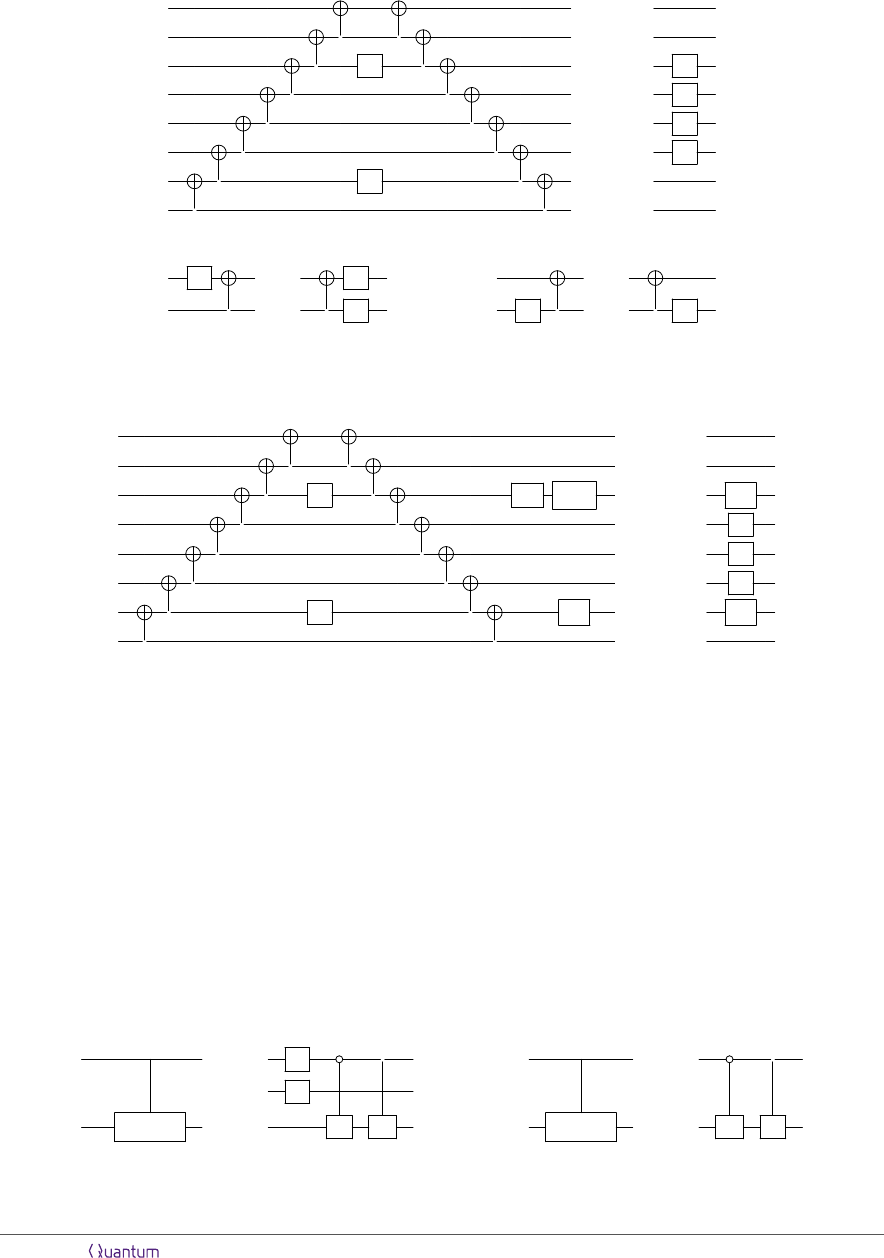To construct a circuit that implements Eq. (4), our starting point is the following circuit identity:
Z
Z
=
Z
Z
Z
Z
(5)
which is an immediate consequence of the elementary identities
Z
=
Z
=
Z Z
Z
and the fact that cnot is self-inverse. The analogue of Eq. (5) for an arbitrary number of qubits
and with the two Z operators on a diﬀerent pair of qubits is obvious. Letting Q
P
1
denote the Pauli
operator such that Q
P
1
Z = iP
1
for P
1
X, ±Y } (i.e., Q
±X
= ±Y and Q
±Y
= X), it follows that
p
Z
i
Q
P
1
p
P
1
=
Z
Z
Z
q
Z
P
2
q
P
2
(6)
Therefore, if the n qubits in the system register are ordered such that the qubit corresponding to
spin-orbital 0 is on the top wire and the qubit corresponding to spin-orbital n1 is on the bottom, the
circuit on the left-hand side would implement the term (P
1
)
p
~
Z
p,q
(P
2
)
q
|ψi for a particular p, q, P
1
, P
2
.
From here, we would obtain a circuit for Select(H) if we were to control the Q
P
1
, P
2
, and Z
operators in the circuit of Eq. (6) on the selection register such that
(1) the states |P
1
i and |P
2
i of the third and fourth selection subregisters determine which Pauli
operators Q
P
1
and P
2
represent, and
(2) conditioned on the ﬁrst two selection subregisters being in the state |pi|qi, Q
P
1
and one of the
Z operators are applied to qubit p of the system register, while P
2
and the other Z operator are
applied to qubit q.
Condition (1) can be straightforwardly satisﬁed by constructing Select(Q) and Select(P ) oper-
ators that choose the appropriate Q
P
1
and P
2
according to the states |P
1
i and |P
2
i. For concreteness,
suppose that |P
1
i = |00i, |01i, |10i, |11i for P
1
= X, X, Y, Y , respectively, and |P
2
i = |0i, |1i for
P
2
= X, Y , respectively. Then, Select(Q) and Select(P ) can be implemented as
/
Z
=
Z
=
Sel(Q)
Y X
Sel(P )
X Y
(7)
Condition (2) requires the ability to target a particular qubit in the system register depending
on the states of the selection subregisters that encode |pi and |qi. For this purpose, we deﬁne for
Accepted in Quantum 2021-01-04, click title to verify. Published under CC-BY 4.0. 5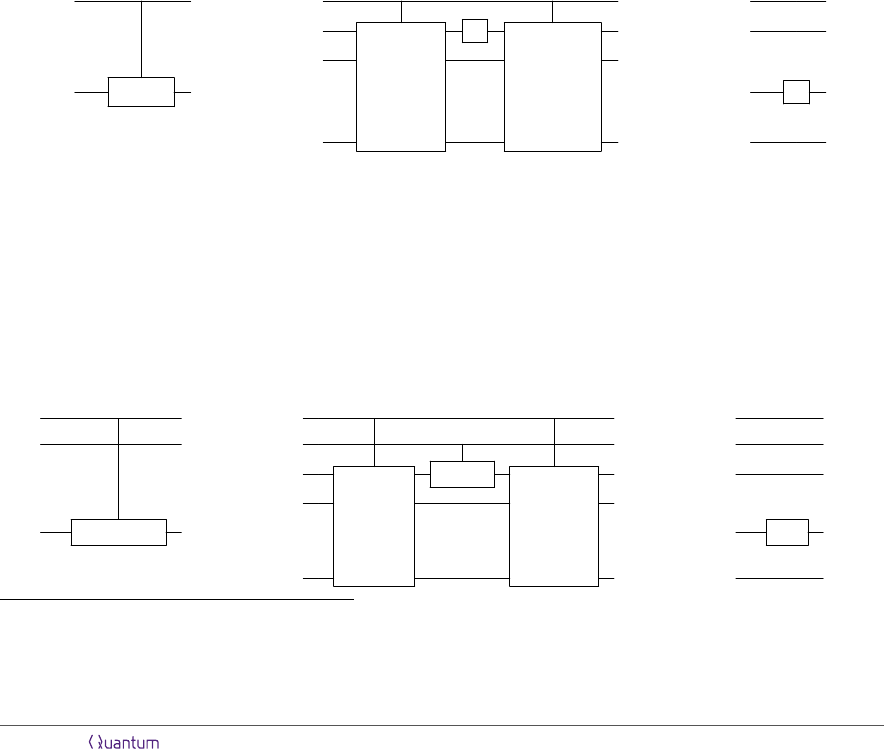any single-qubit unitary U a (dlog ne + n)-qubit operator Inject(U). When applied to |xi|ψi, where
x {0, . . . , n 1} (encoded in binary) and |ψi is an arbitrary n-qubit state, Inject(U ) implements
U on qubit x of |ψi and acts as the identity on the other qubits, i.e.,
Inject(U) : |xi|ψi 7→ |xi
h
I
x
U I
(nx1)
|ψi
i
.
To synthesise Inject(U) for any U, we use a (dlog ne + n)-qubit operator SwapUp, deﬁned as follows.
For any x {0, . . . , n 1} and n-qubit product state
N
n1
y=0
|ϕ
y
i
y
,
SwapUp : |xi
n1
O
y=0
|ϕ
y
i
y
7→ |xi|ϕ
x
i
0
n1
O
y=1
|ϕ
σ(y)
i
y
, (8)
where σ is a ﬁxed
3
permutation of {0, . . . , n 1} such that σ(0) = x. In other words, conditioned
on the dlog ne-qubit control register being in the state x for x {0, . . . , n 1}, SwapUp moves the
state |ϕ
x
i of the qubit indexed by x in the target register up to the ﬁrst qubit of the target register,
and permutes the states of the other qubits in some way. Ref.  shows that SwapUp can be
implemented without ancilla qubits using O(n) Cliﬀord and T gates, O(log
2
n) Cliﬀord-depth, and
O(log n) T -depth. We sketch the construction in Appendix A.1. From Eq. (8), it is easy to see that
Inject(U) = SwapUp
(U I
(n1)
)SwapUp. SwapUp permutes the qubits in the target register in
such a way that the state of qubit x is moved up to the ﬁrst qubit, U is then applied to the ﬁrst qubit,
and SwapUp
undoes the permutation.
|xi /
|xi /
|xi /
=
SwapUp
U
SwapUp
=
0
.
.
.
|ψi / Inj(U)
|ψi
.
.
.
x
U
.
.
.
n 1
(9)
(The third circuit above illustrates the eﬀect of Inject(U ) in the special case where the input to the
control register is a computational basis state, whereas the second circuit implements Inject(U ) on
arbitrary inputs.)
Hence, we can ensure that the two Z operators in Eq. (6) are applied to qubits p and q of the system
register conditioned on the state of the ﬁrst two selection subregisters being |pi|qi by implementing
two Inject(Z) operators, one with |pi as the control register and the other with |qi as the control
register. To correctly apply Q
P
1
and P
2
, we use the Select(Q) and Select(P ) circuits constructed
in Eq. (7) in conjunction with SwapUp to form Inject-Select(Q) and Inject-Select(P ). This is
shown below for Inject-Select(Q); the construction of Inject-Select(P ) is analogous.
|pi /
|pi /
|pi /
|P
1
i /
|P
1
i /
|P
1
i /
=
SwapUp
Sel(Q)
SwapUp
=
0
.
.
.
|ψi /
Inj-Sel(Q)
|ψi
.
.
.
p
Q
P
1
.
.
.
n 1
(10)
3
In principle, any permutation σ such that σ(0) = x would work—the action of σ on {1, . . . , n 1} is not relevant
to the correctness of our construction. However, the implementation of SwapUp in Appendix A.1 yields a particular
permutation σ (for each n).
Accepted in Quantum 2021-01-04, click title to verify. Published under CC-BY 4.0. 6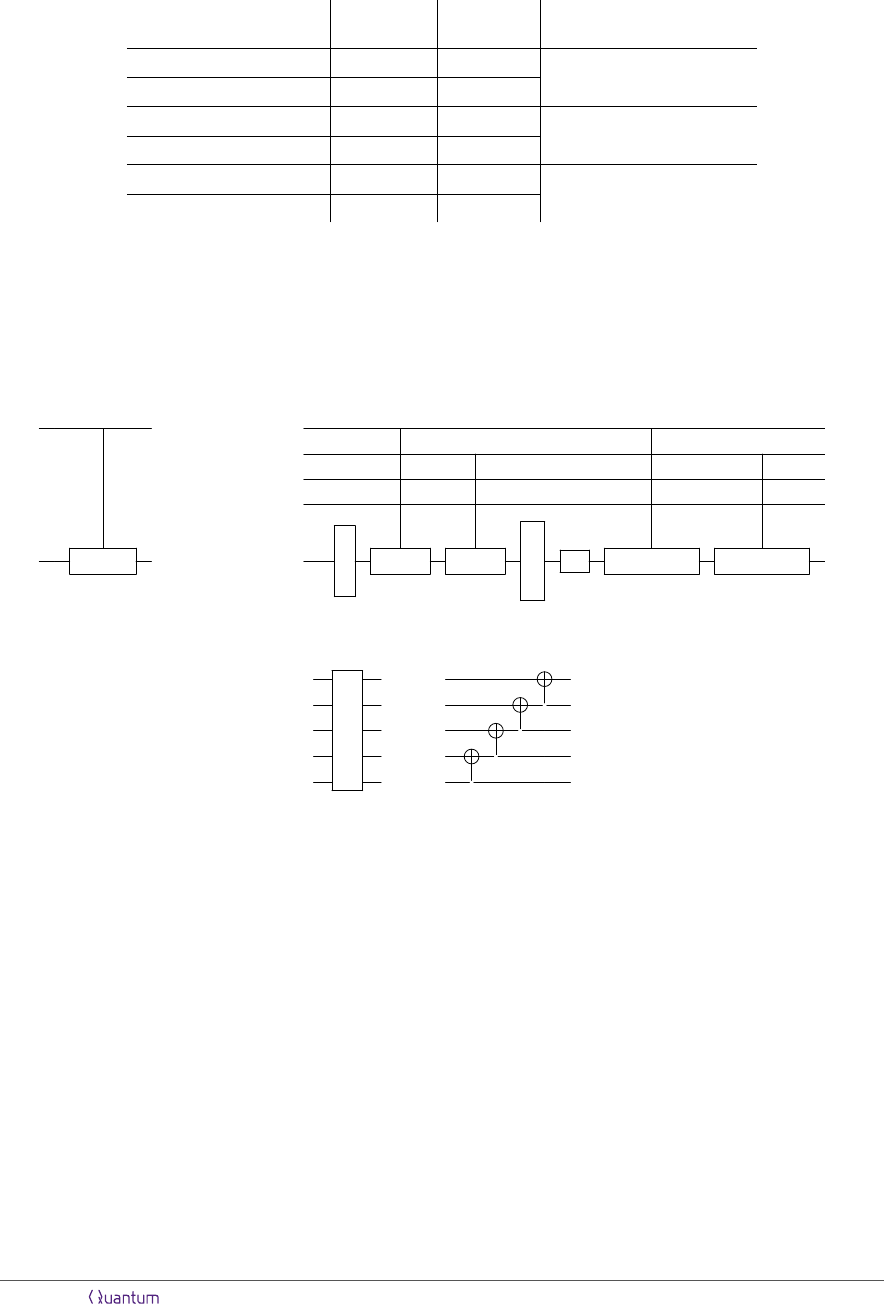circuit component T -count T -depth
# of elementary gates
to control
Inject(Z) 28(n 1) 32dlog ne
1
Inject
(Z) 8(n 1) 8dlog ne
Inject-Select(Q) 28(n 1) 32dlog ne
4
Inject-Select
(Q) 16(n 1) 16dlog ne
Inject-Select(P ) 28(n 1) 32dlog ne
2
Inject-Select
(P ) 16(n 1) 16dlog ne
Table 1: T -count and T -depth of each of the main components used to construct circuits for Select(H) in
Section 2. (The Cliﬀord-count and Cliﬀord-depth are O(n) and O(log
2
n), respectively, for all of the components.)
The fourth column speciﬁes the number of one- or two-qubit (Cliﬀord) gates to which controls need to be added in
order to construct the controlled version of the operator in the ﬁrst column.
With these components in hand, we can assemble the circuit for Select(H):
|i /
|pi /
|qi /
=
|i
|P
1
i /
|P
2
i
|ψi / Sel(H) |ψi /
Inj(Z) Inj(Z)
i
Inj-Sel(Q) Inj-Sel(P )
(11)
where Ladder denotes the operator implemented by the ladder-like sequence of n 1 cnot gates in
Eq. (6):
:
=
(12)
By comparing the circuit in Eq. (11) to that in Eq. (6), it can be veriﬁed that the former correctly
implements Select(H) as it is deﬁned in Eq. (4). When the selection register is in the computational
basis state |pi|qi|P
1
i|P
2
i, the two Inject(Z) operators apply Z operators on qubits p and q in the
system register, between the two sequences of cnot gates corresponding to Ladder and Ladder
.
Then, Inject-Select(Q) applies Q
P
1
to qubit q and Inject-Select(P ) applies P
2
to qubit q. Thus,
by Eq. (6), the circuit applies the operator (P
1
)
p
~
Z
p,q
(P
2
)
q
on the system register conditioned on the
state of the selection register being |pi|qi|P
1
i|P
2
i, as required by Eq. (4). This holds for all of the
computational basis states, and therefore for arbitrary states of the selection register.
It is clear from Eqs. (7), (9), and (10) that the only non-Cliﬀord gates in the circuit of Eq. (11) are
the SwapUp gadgets used to construct the Inject operators. As shown in Appendix A.1, SwapUp
on dlog ne+n qubits can be implemented with O(n) T gates and O(log n) T -depth. Each of Inject(Z),
Inject-Select(Q), and Inject-Select(P ) uses one SwapUp and one SwapUp
. Since the number
of these operators is a constant independent of n, the total T -count of the circuit in Eq. (11) is O(n)
and the total T -depth is O(log n). The exact T costs of the components are provided by Table 1.
The Cliﬀord complexity of Inject(Z), Inject-Select(Q), and Inject-Select(P ) is dominated
by that of SwapUp and its inverse, which have Cliﬀord-count O(n) and Cliﬀord-depth O(log
2
n)
[cf. Appendix A.1]. The circuit for the Ladder operator given in Eq. (12) has Cliﬀord-depth n 1;
however, as shown in Appendix A.3, the same operator can be implemented using an ancilla-free circuit
Accepted in Quantum 2021-01-04, click title to verify. Published under CC-BY 4.0. 7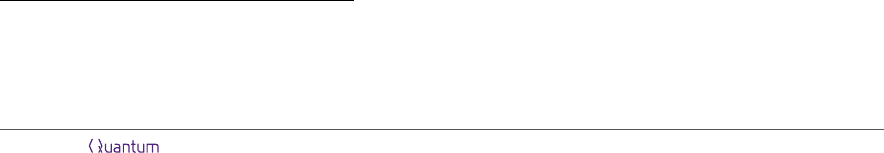consisting of O(n) cnots arranged in O(log n) layers. It follows that the total Cliﬀord-count of the
circuit in Eq. (11) is O(n) and the total Cliﬀord-depth is O(log
2
n).
Although the circuit of Eq. (11) achieves O(n) T -count and O(log n) T -depth, we can reduce the
constant factors hidden under the big O if desired by making a few modiﬁcations, as demonstrated
in the following subsection. We conclude this subsection by noting that the controlled version of
Select(H) can be constructed by adding controls to a very small number of gates in the circuit—
namely, the Z operator in each Inject(Z) [cf. Eq. (9)], the Pauli and controlled-Pauli operators
in Inject-Select(Q) and Inject-Select(P ) [cf. Eqs. (7) and (10)], and the (i)-phase gate (by
implementing an S
gate on the control qubit). When these operators are not applied, the circuit
implements the identity since the Ladder and SwapUp operators are cancelled by their inverses.
Therefore, controlling the entire circuit on any constant number of qubits incurs only constant additive
gate complexity (which can be quantiﬁed using the fourth column of Table 1). This is important
because algorithms that use the LCU query model generally require access to controlled-Select(H).
2.2 Reducing the constant factors
Before generalising the Select(H) circuit in subsection 2.1 to arbitrary fermionic Hamiltonians, we
provide more eﬃcient versions of the main circuit components, which can be used to reduce the T -count
and T -depth by constant multiplicative factors. The Cliﬀord-count and Cliﬀord-depth are reduced by
constant factors as well. However, we focus on the T complexity in this subsection because T gates are
the bottleneck in many models of fault-tolerant quantum computation, notably those that are based
on topological error correcting codes. In these settings, T gates require signiﬁcantly more time and
physical qubits to implement than Cliﬀord gates , and even a constant-factor improvement in the
T complexity may be useful.
The strategy is to replace all of the SwapUp operators by a particular phase-incorrect SwapUp
operator, which is based on a phase-incorrect Toffoli gate introduced in Ref. . As pointed out
by Ref. , this phase-incorrect SwapUp, which we will call SwapUp
, can be implemented using
4(n1) T gates that are applied in 4dlog ne layers, a considerable reduction from the 14(n1) T -count
and 16dlog ne T -depth of SwapUp. The circuit for SwapUp
is described in Appendix A.2. In the
computational basis, SwapUp
has the same matrix elements as SwapUp up to sign, i.e., for any
computational basis state |zi,
SwapUp
|zi = ±SwapUp|zi = ±|z
0
i,
where |z
0
i = SwapUp|zi is also a computational basis state. Hence, we can write SwapUp
=
D · SwapUp for some operator D that is diagonal in the computational basis, with eigenvalues ±1.
This implies that Inject(Z) would still be implemented correctly if SwapUp and SwapUp
in the
circuit of Eq. (9) were replaced with SwapUp
and SwapUp
∗†
:
SwapUp
∗†
Z
1
SwapUp
= (SwapUp
D
)Z
1
(D · SwapUp)
= SwapUp
Z
1
SwapUp = Inject(Z),
where the second equality follows from the fact that D commutes with Z
1
and is unitary. We denote
this implementation of Inject(Z) by Inject
(Z).
4
By the same token, SwapUp
can be used to
construct Inject(U) for any U that is diagonal in the computational basis.
On the other hand, the circuit in Eq. (10) would not correctly implement Inject-Select(Q) if
SwapUp
were used instead of SwapUp, since X and Y are not diagonal in the computational basis.
To reduce the T cost of Inject-Select(Q), we modify the circuits so that X and Y are “injected”
4
We clarify that unlike in the case of SwapUp and SwapUp
, which are diﬀerent operators, Inject(Z) and
Inject
(Z) designate diﬀerent circuit implementations of the same operator. The same goes for Inject-Select(Q)
and Inject-Select
(Q), and Inject-Select(P ) and Inject-Select
(P ).
Accepted in Quantum 2021-01-04, click title to verify. Published under CC-BY 4.0. 8separately by applying Inject
(Z) conjugated by basis change operators, as follows:
|pi /
|pi /
|P
1
i /
=
|P
1
i
Z
Z
|ψi / Inj-Sel
(Q) |ψi /
C
Y
Inj
(Z)
C
Y
C
X
Inj
(Z)
C
X
using C
X
and C
Y
to represent B
n
X
and B
n
X
, where B
X
and B
Y
are Cliﬀord gates for which
B
X
ZB
X
= X and B
Y
ZB
Y
= Y . Note that controlled-Inject(Z) can be constructed by sim-
ply adding a control to the Z operator in Inject
(Z). The construction for Inject-Select(Q)
is similar [cf. Eq. (7)]. We denote these alternative implementations of Inject-Select(Q) and
Inject-Select(P ) by Inject-Select
(Q) and Inject-Select
(P ).
The T -count and T -depth of each of these improved circuit components follow directly from the T
cost of SwapUp
, and are listed in Table 1. Inject(Z), Inject-Select(P ), and Inject-Select(Q)
can always be replaced with their asterisked counterparts to minimise the complexity. For example, the
circuit in Eq. (11), which implements Select(H) for quadratic fermionic Hamiltonians, has T -count
112(n 1) and T -depth 128dlog ne. Replacing the components in Eq. (11) by their improved versions
would reduce the total T -count to 48(n 1) and the T -depth to 48dlog ne.
5
2.3 Generalising to arbitrary k
We can extend the ideas of subsections 2.1 and 2.2 to devise ancilla-free implementations of Select(H)
for the Jordan-Wigner transforms of arbitrary fermionic Hamiltonians. If at most k distinct spin-
orbitals are involved in each term of the fermionic Hamiltonian, the resulting circuit has Cliﬀord- and
T -count O(kn), Cliﬀord-depth O(k log
2
n), and T -depth O(k log n). To help illustrate the concepts by
way of circuit diagrams, we will use k = 4 Hamiltonians as a concrete example.
In its second-quantised representation, each term in a general fermionic Hamiltonian is a product of
interaction operators a
p
a
q
or a
p
a
q
(with p < q) and their Hermitian conjugates, and number operators
n
p
:
= a
p
a
p
.
6
By deﬁnition of k, Hamiltonians with k = 4 may include such terms as a
p
a
q
a
r
a
s
+ h.c.,
a
p
a
q
n
r
n
s
+h.c., n
p
, and n
p
n
q
n
r
, to list a few examples. The circuit for Select(H) can be constructed
in two main parts. Loosely speaking, one part of the circuit implements interaction operators and the
other part implements number operators.
As we saw in subsection 2.1, an interaction operator involving two spin-orbitals p and q is mapped
to linear combinations of Pauli “strings” of the form (P
1
)
p
~
Z
p,q
(P
2
)
q
under the Jordan-Wigner trans-
formation , with P
1
, P
2
{X, Y } [cf. Eqs. (2) and (3)]. More generally, any product of interac-
tion operators is mapped to a linear combination of products of such Pauli strings. For instance,
a
p
a
q
a
r
a
s
+ h.c. (for p < q < r < s) becomes a linear combination of (P
1
)
p
~
Z
p,q
(P
2
)
q
(P
3
)
r
~
Z
r,s
(P
4
)
q
,
for P
1
, P
2
, P
3
, P
4
{X, Y }. Hence, the circuit in Eq. (11), which implements Select(H) in the spe-
cial case that the Hamiltonian consists only of interactions between two spin orbitals, can be easily
expanded to implement Hamiltonians containing arbitrary products of interaction operators. The key
observation is that the identities in Eqs. (5) and (6) hold analogously for any number of pairs of Z
5
As a side note, the Toffoli-count of any of the non-asterisked components can be obtained by dividing the corre-
sponding T -count in Table 1 by 7, and the Toffoli-depth can be obtained by dividing the T -depth by 4. The asterisked
operators are not constructed using Toffolis. See Appendix A.1 and A.2 for details.
6
In theory, the Hamiltonian could contain terms that are linear, cubic, etc. in the fermionic operators. Our method
can be used to implement these terms as well (basically, by exploiting the identity in Eq. (5) except with an odd number
of Z operators), but since they rarely appear in Hamiltonians of interest, we omit them for simplicity.
Accepted in Quantum 2021-01-04, click title to verify. Published under CC-BY 4.0. 9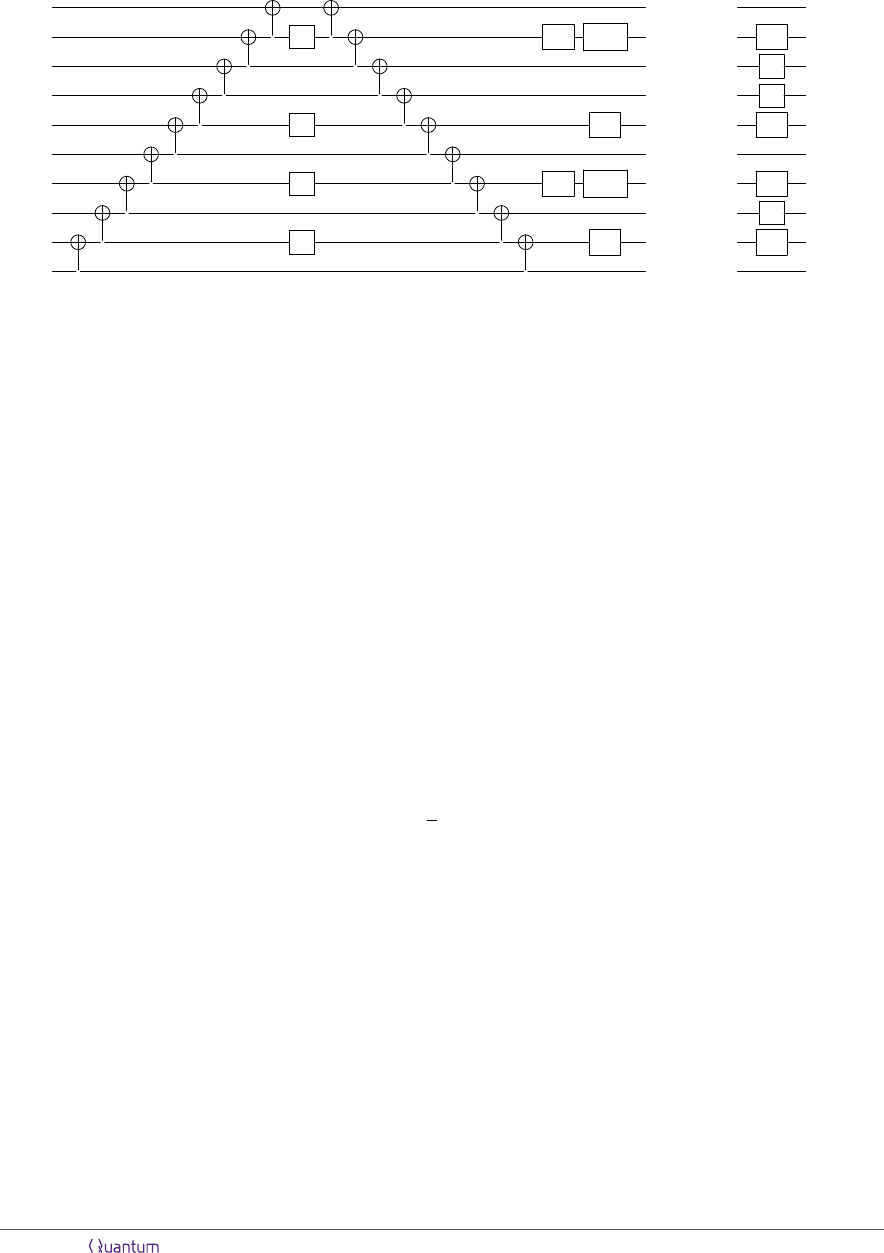operators, e.g., for two pairs of Z operators, we have
p
Z
i
Q
P
1
p
P
1
Z
Z
q
Z
P
2
=
q
P
2
r
Z
i
Q
P
3
r
P
3
Z
s
Z
P
4
s
P
4
(13)
Consequently, by the exact same logic as that in subsection 2.1, we can “select” between Pauli operators
corresponding to interaction terms using a circuit composed of the Inject(Z), Inject-Select(Q),
and Inject-Select(P ) subroutines deﬁned in subsection 2.1, along with a Ladder and Ladder
.
This circuit would essentially be an extended version of that in Eq. (11), with two minor modiﬁcations.
First, instead of absorbing the sign of the Pauli operator into P
1
, as we did in subsection 2.1, we use
one qubit |sgni in the selection register to encode the sign (with |sgni = |0i corresponding to +1 and
|sgni = |1i to 1). We then remove the second wire in the circuit for Select(Q) in Eq. (7), and
modify Inject-Select(Q) accordingly. Second, to account for the possibility that diﬀerent terms in
the Hamiltonian may be products of diﬀerent numbers of interaction operators (e.g., a
p
a
q
+ h.c. and
a
p
a
q
a
r
a
s
+ h.c. may both be present in a k = 4 Hamiltonian), we use k of the qubits in the selection
register as control qubits. We denote the states of these control qubits by |i
p
i, |i
q
i, |i
r
i, |i
s
i, etc.
Note that the Pauli string (P
3
)
r
~
Z
r,s
(P
4
)
s
on the right-hand side of Eq. (13) would not be implemented
if the bottom two Z operators, Q
P
3
, and P
4
are not applied on the left-hand side. It follows that
by controlling the corresponding Inject(Z), Inject-Select(P ), and Inject-Select(Q) operators
on |i
r
i and |i
s
i, either (P
1
)
p
~
Z
p,q
(P
2
)
q
(P
3
)
r
~
Z
r,s
(P
4
)
q
or (P
1
)
p
~
Z
p,q
(P
2
)
q
is applied depending on the
state |i
r
i|i
s
i. The operators associated with p and q are controlled as well in order to allow for the
implementation of number operators, which do not transform into Pauli strings of the form in Eq. (13)
[cf. Eq. (14) below]. For the example of k = 4, the (sub)circuit for interaction operators is the left part
(labelled “interaction operators”) of the circuit in Eq. (15).
Number operators are very straightforward to implement using Inject(Z) gates, since
n
p
7→
1
2
(I Z
p
) (14)
under the Jordan-Wigner transformation. Therefore, to incorporate the Hamiltonian terms that involve
number operators, the state of the selection register simply needs to indicate whether Z operators
should be applied on certain qubits (recalling that the overall sign is encoded in |sgni). It suﬃces to
use another k of the selection register qubits as control qubits, labelling their states by |n
p
i, |n
q
i, |n
r
i,
|n
s
i, etc., and control an Inject(Z) operator on |pi and |n
p
i, another Inject(Z) operator on |qi and
|n
q
i, and so on. The full circuit for Select(H) for any k = 4 Hamiltonian is shown in Eq. (15) below.
As always, each of the Inject(Z), Inject-Select(Q), and Inject-Select(P ) gates can be replaced
by their more eﬃcient variants constructed in subsection 2.2.
All possible terms can be encoded by appropriately choosing the correspondence between the Pauli
operators in the Jordan-Wigner encoding and the computational basis states of each subregister (and
constructing Prepare(α) in a way that is consistent with this correspondence). As an explicit example,
suppose that the Hamiltonian includes terms of the form a
p
a
q
n
r
+ h.c., which transform to linear
combinations of (P
1
)
p
~
Z
p,q
(P
2
)
q
and (P
1
)
p
~
Z
p,q
(P
2
)
q
Z
r
, with P
1
, P
2
{X, Y }. It can be seen from
Eq. (15) that the ﬁrst type of Pauli operators are applied when |n
r
i = |0i and the second type are
Accepted in Quantum 2021-01-04, click title to verify. Published under CC-BY 4.0. 10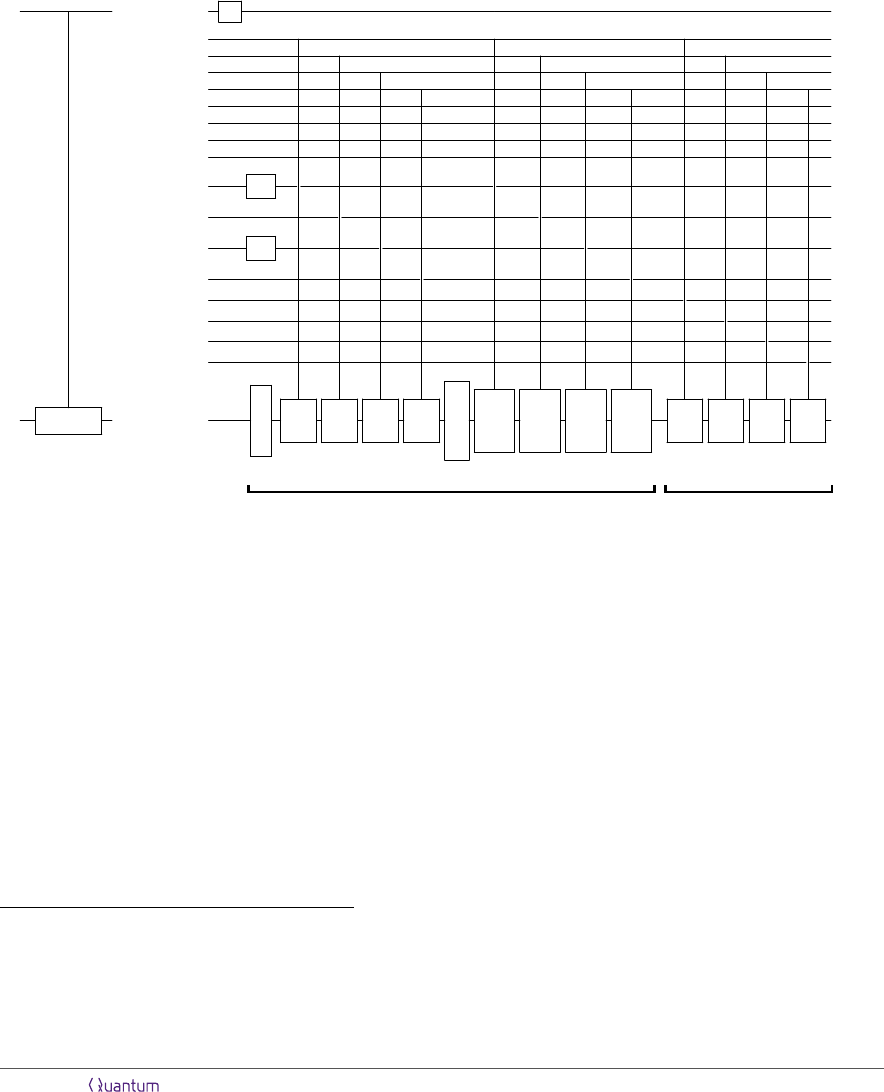applied when |n
r
i = |1i, with |i
p
i|i
q
i|i
r
i|i
s
i = |1i|1i|0i|0i and |n
p
i|n
q
i|n
s
i = |0i|0i|0i for both. While
the circuit in Eq. (15) implements arbitrary terms involving up to k = 4 spin-orbitals, it is often the
case that the Hamiltonian in question only contains a few types of terms. Some of the qubits could then
be removed from the selection register and the control logic could be simpliﬁed. For the molecular
electronic structure Hamiltonian, which is a linear combination of a
p
a
q
+ h.c., a
p
a
q
a
r
a
s
+ h.c., n
p
,
n
p
n
q
, and a
p
a
q
n
r
+ h.c. [34, 35], we would not need the qubits storing |n
r
i and |n
s
i and the last two
Inject(Z) gates in Eq. (15), for instance.
|`i /
|sgni
Z
|pi /
|qi /
|ri /
|si /
|P
1
i
|P
2
i
|P
3
i
|P
4
i
=
|i
p
i
S
|i
q
i
|i
r
i
S
|i
s
i
|n
p
i
|n
q
i
|n
r
i
|n
s
i
|ψi / Sel(H) |ψi /
Inj
(Z)
Inj
(Z)
Inj
(Z)
Inj
(Z)
Inj-
Sel
(Q)
Inj-
Sel
(P )
Inj-
Sel
(Q)
Inj-
Sel
(P )
Inj
(Z)
Inj
(Z)
Inj
(Z)
Inj
(Z)
interaction operators
number operators
(15)
Thus, for arbitrary k, the circuit for Select(H) can be constructed from at most 2k controlled-
Inject(Z), bk/2c controlled-Inject-Select(Q), and bk/2c controlled-Inject-Select(P), and 2
Ladder operators. Inject(Z), Inject-Select(Q), and Inject-Select(P ) are implemented us-
ing O(n) Cliﬀord and T gates, O(log
2
n) Cliﬀord-depth, and O(log n) T -depth, while Ladder can be
implemented using O(n) Cliﬀord gates and O(log n) Cliﬀord-depth [cf. Appendix A.3]. The circuit
therefore has Cliﬀord- and T -count O(kn), Cliﬀord-depth O(k log
2
n), and T -depth O(k log n) in all
cases. It is clear from Eq. (15) that the selection register comprises kdlog ne + O(1) qubits, and that
no ancillae are required. For practical applications, k is usually a constant independent of n, in which
case the total gate count is O(n) and the Cliﬀord-depth and T -depth are O(log
2
n) and O(log n),
respectively. The exact constant factors
7
in the T complexity can be directly calculated using Table 1.
Acknowledgments
The author is grateful to Isaac Kim for mentorship and helpful discussions, and to Ryan Babbush,
Earl Campbell, and Craig Gidney for insightful comments on an earlier draft.
7
As a comparison to previous work, the implementations of controlled-Select(H) in Ref.  for the molecular
electronic structure Hamiltonian and for the planar Fermi-Hubbard Hamiltonian have T -counts of 12n + O(log n) and
10n + O(log n), respectively, and T -depth O(n). In our framework, controlled-Select(H) can be implemented for both
Hamiltonians using the same circuit, and this circuit would have T -count 64n + O(1) and T -depth 64dlog ne + O(1).
Hence, the exponential improvement in overall circuit depth comes at the cost of only a modest constant-factor increase
in the T -count.
Accepted in Quantum 2021-01-04, click title to verify. Published under CC-BY 4.0. 11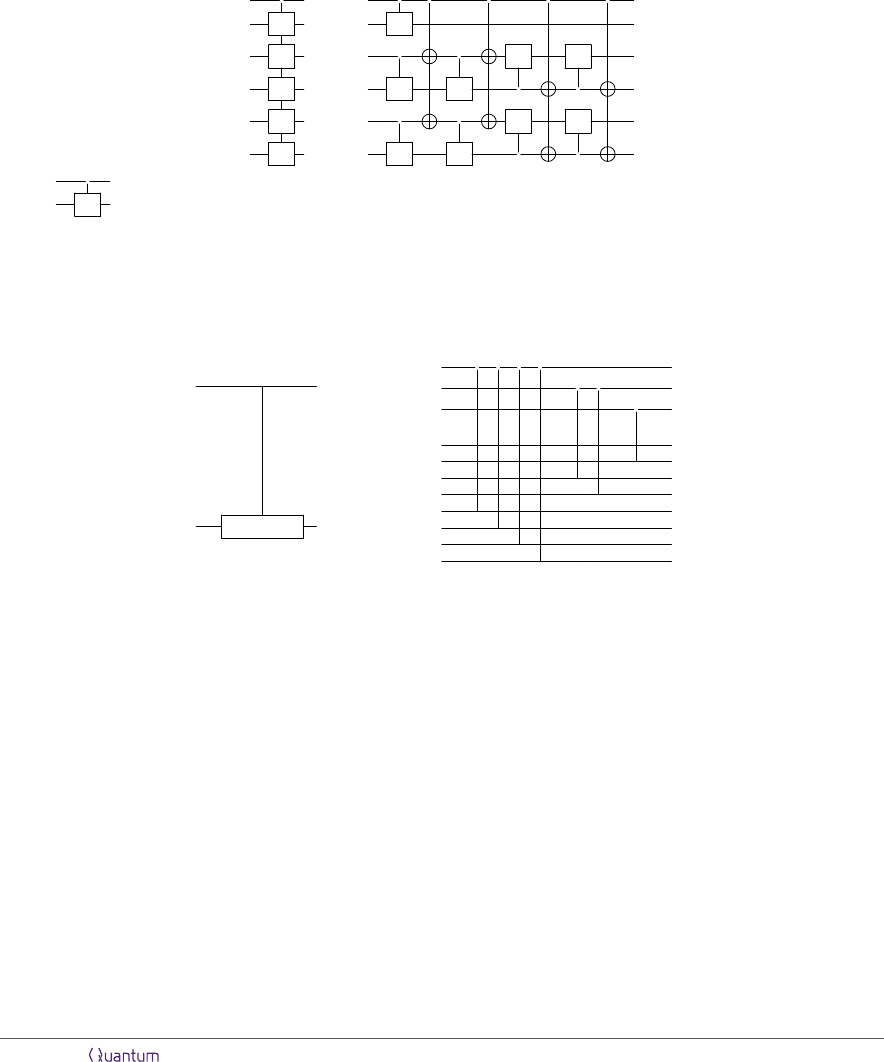A.1 SwapUp
Ref.  provides an ancilla-free, logarithmic-depth circuit for the SwapUp operator deﬁned by Eq. (8).
As the ability to implement SwapUp using only O(log
2
n) Cliﬀord-depth and O(log n) T -depth is
essential for proving our main result, we summarise the construction here.
First, Ref.  observes that for any self-inverse unitary V (on any number of qubits), the multi-
target controlled-V gate c(V )
m
:
= |0ih0| I + |1ih1| V
m
on m registers can be implemented by a
circuit of the form
/
V
/
V
/
V
=
/
V V
/
V
/
V V
/
V
/
V V
/
V
/
V V
(16)
where
/
/
V
indicates that a controlled-V gate is applied with any one of the qubits in the ﬁrst register
as the control qubit. (If m is even, the second wire and the topmost controlled-V gate in Eq. (16) are
removed.) Thus, c(V )
m
can be implemented without ancilla qubits using at most 2m controlled-V
gates and O(m) cnots in such a way that the controlled-V gates are applied in 4 layers. Ref. 
further shows that the multi-target cnots can be implemented in depth O(log m).
Next, consider the following circuit for SwapUp, drawn for n = 8:
|xi /
=
7
N
y=0
|ϕ
y
i
/
SwapUp
|x
2
i
|x
1
i
|x
0
i
|ϕ
0
i × × × |ϕ
x
i
|ϕ
1
i × × ×
|ϕ
2
i × ×
|ϕ
3
i × ×
|ϕ
4
i ×
|ϕ
5
i ×
|ϕ
6
i ×
|ϕ
7
i ×
(17)
Here, x
2
x
1
x
0
is the binary representation of x, where x
0
is the least signiﬁcant bit. The basic idea is
that after the controlled-swap gates controlled on the qubit storing |x
2
i are applied, the states |ϕ
y
i
with y
2
= x
2
are on the ﬁrst four qubits of the second register (writing y in binary as y
2
y
1
y
0
). After
the controlled-swaps controlled on |x
1
i are applied, the states |ϕ
y
i with y
2
= x
2
and y
1
= x
1
are on
the ﬁrst two qubits, and ﬁnally the controlled-swap controlled on |x
0
i ensures that |ϕ
x
i ends up on the
very ﬁrst qubit. In general, if the target register has n qubits (where n is not necessarily a power of 2)
and x
dlog ne−1
. . . x
1
x
0
is the binary representation of x, n 2
dlog ne−1
controlled-swaps are controlled
on |x
dlog n1e
i, followed by 2
j
controlled-swaps controlled on |x
j
i for each j {0, . . . , dlog ne 2} in
descending order.
Note that for each j {0, . . . , dlog ne 1}, the controlled-swap gates controlled on |x
j
i target
disjoint pairs of qubits. Since swap is self-inverse, we can apply Eq. (16) with V = swap and m 2
j
(speciﬁcally, m = 2
j
for j {0, . . . , dlog ne 2} and m = n 2
dlog ne−1
for j = dlog ne 1) to replace
all of the controlled-swaps controlled on |x
j
i in Eq. (17) with at most 2 · 2
j
controlled-swaps applied
in 4 layers, along with O(2
j
) cnots applied in O(j) layers. Summing over j, the total number of
controlled-swaps is
2
n 2
dlog ne−1
+
dlog ne−2
X
j=0
2 · 2
j
= 2(n 1)
Accepted in Quantum 2021-01-04, click title to verify. Published under CC-BY 4.0. 12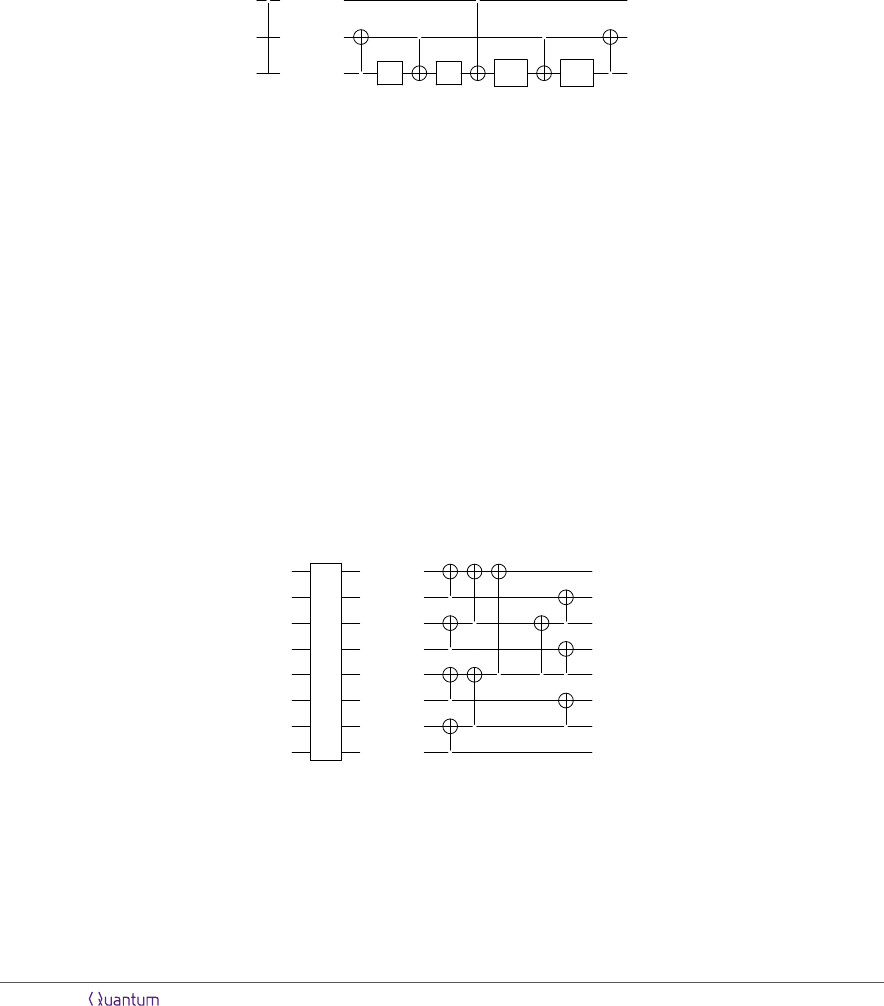and these are applied in 4dlog ne layers. Each controlled-swap can be decomposed into one Toffoli
and two cnot gates, and each Toffoli has T -count 7 and T -depth 4. Therefore, SwapUp has T -
count 14(n 1) and T -depth 16dlog ne. The total number of Cliﬀord gates (including the multi-target
cnots in Eq. (16) and the Cliﬀords in the decomposition of each controlled-swap) is O(n), and the
total Cliﬀord-depth is O(log
2
n).
A.2 SwapUp
In subsection 2.2, we use the fact that a certain phase-incorrect version SwapUp
of SwapUp is less
costly than SwapUp to reduce the T -count and T -depth by constant multiplicative factors. SwapUp
is obtained by replacing each controlled-swap gate in Eq. (17) with a phase-incorrect version of
controlled-swap:
×
×
A A
A
A
where A
:
= e
i(π/8)Y
= S
HTHS (here, H denotes the Hadamard gate). The circuit on the right-
hand side implements an operator whose action on computational basis states diﬀers from controlled-
swap only in that |100i is mapped to −|100i. Hence, since controlled-swap merely permutes the
computational basis states, SwapUp
acts the same as SwapUp on computational basis states up to
sign. As observed in Ref. , any sequence of these phase-incorrect controlled-swap operators that
target disjoint pairs of qubits can be parallelised such that the A and A
gates are applied in four
layers, and the cnots in O(log n) layers. It follows that SwapUp
has T -count 4(n 1) and T -depth
4dlog ne (and Cliﬀord-count O(n) and Cliﬀord-depth O(log
2
n)).
The Ladder operator, deﬁned in Eq. (12) by a cascade of n 1 cnot gates, can equivalently be
implemented using a logarithmic-depth circuit, as pointed out by Gidney . By Eq. (12), Ladder
acts on computational basis states as
0
i|z
1
i . . . |z
n1
i 7→ |z
0
· · · z
n1
i|z
1
· · · z
n1
i . . . |z
n1
i.
It is easily veriﬁed that the same transformation can be realised by arranging cnot gates in a tree-like
structure, shown below for n = 8:
=
(For n that is not a power of 2, the circuit for Ladder can be obtained by starting with the circuit
for the next largest power of 2, then removing all of the cnots supported on qubits that are out of
range.) Thus, a circuit Ladder can be constructed using O(n) cnots applied in O(log n) layers.
Accepted in Quantum 2021-01-04, click title to verify. Published under CC-BY 4.0. 13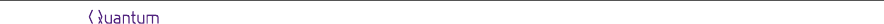References
 Sam McArdle, Suguru Endo, Alán Aspuru-Guzik, Simon C. Benjamin, and Xiao Yuan. Quan-
tum computational chemistry. Reviews of Modern Physics, 92(1), 2020. DOI: 10.1103/RevMod-
Phys.92.015003.
 Yudong Cao, Jonathan Romero, Jonathan P. Olson, Matthias Degroote, Peter D. Johnson, Mária
Kieferová, Ian D. Kivlichan, Tim Menke, Borja Peropadre, Nicolas P. D. Sawaya, Sukin Sim, Libor
Veis, and Alán Aspuru-Guzik. Quantum chemistry in the age of quantum computing. Chemical
Reviews, 119(19):10856–10915, 2019. DOI: 10.1021/acs.chemrev.8b00803.
 Bela Bauer, Sergey Bravyi, Mario Motta, and Garnet Kin-Lic Chan. Quantum algorithms for
quantum chemistry and quantum materials science. Chemical Reviews, 120(22):12685–12717,
2020. DOI: 10.1021/acs.chemrev.9b00829.
 Daniel S. Abrams and Seth Lloyd. Quantum algorithm providing exponential speed increase
for ﬁnding eigenvalues and eigenvectors. Physical Review Letters, 83(24):5162–5165, 1999. DOI:
10.1103/PhysRevLett.83.5162.
 A. Yu. Kitaev. Quantum measurements and the abelian stabilizer problem, 1995. URL https:
//arxiv.org/abs/quant-ph/9511026.
 R. Cleve, A. Ekert, C. Macchiavello, and M. Mosca. Quantum algorithms revisited. Proceedings
of the Royal Society of London. Series A: Mathematical, Physical and Engineering Sciences, 454
(1969):339–354, 1998. DOI: 10.1098/rspa.1998.0164.
 R. D. Somma, S. Boixo, H. Barnum, and E. Knill. Quantum simulations of classical annealing
processes. Physical Review Letters, 101(13), 2008. DOI: 10.1103/PhysRevLett.101.130504.
 David Poulin, Alexei Kitaev, Damian S. Steiger, Matthew B. Hastings, and Matthias Troyer.
Quantum algorithm for spectral measurement with a lower gate count. Physical Review Letters,
121(1), 2018. DOI: 10.1103/PhysRevLett.121.010501.
 Edward Farhi, Jeﬀrey Goldstone, Sam Gutmann, and Michael Sipser. Quantum computation by
 Dorit Aharonov and Amnon Ta-Shma. Adiabatic quantum state generation and statistical zero
knowledge, 2003. URL https://arxiv.org/abs/quant-ph/0301023.
 Guang Hao Low and Isaac L. Chuang. Optimal Hamiltonian simulation by quantum signal pro-
cessing. Physical Review Letters, 118(1), 2017. DOI: 10.1103/PhysRevLett.118.010501.
 Guang Hao Low and Isaac L. Chuang. Hamiltonian simulation by qubitization. Quantum, 3:163.
DOI: 10.22331/q-2019-07-12-163.
 Dominic W. Berry, Mária Kieferová, Artur Scherer, Yuval R. Sanders, Guang Hao Low, Nathan
Wiebe, Craig Gidney, and Ryan Babbush. Improved techniques for preparing eigenstates of
fermionic Hamiltonians. npj Quantum Information, 4(1). DOI: 10.1038/s41534-018-0071-5.
 Ryan Babbush, Craig Gidney, Dominic W. Berry, Nathan Wiebe, Jarrod McClean, Alexandru
Paler, Austin Fowler, and Hartmut Neven. Encoding electronic spectra in quantum circuits with
linear T complexity. Physical Review X, 8(4). DOI: 10.1103/PhysRevX.8.041015.
 Guang Hao Low and Nathan Wiebe. Hamiltonian simulation in the interaction picture, 2018.
URL https://arxiv.org/abs/1805.00675.
 Mária Kieferová, Artur Scherer, and Dominic W. Berry. Simulating the dynamics of time-
dependent Hamiltonians with a truncated Dyson series. Physical Review A, 99(4), 2019. DOI:
10.1103/PhysRevA.99.042314.
 Kianna Wan and Isaac Kim. Fast digital methods for adiabatic state preparation, 2020. URL
https://arxiv.org/abs/2004.04164.
 Matthew B. Hastings. Lieb-Schultz-Mattis in higher dimensions. Phys. Rev. B, 69:104431, 2004.
DOI: 10.1103/PhysRevB.69.104431.
 Yimin Ge, Jordi Tura, and J. Ignacio Cirac. Faster ground state preparation and high-precision
ground energy estimation with fewer qubits. Journal of Mathematical Physics, 60(2):022202, 2019.
DOI: 10.1063/1.5027484.
Accepted in Quantum 2021-01-04, click title to verify. Published under CC-BY 4.0. 14Lin Lin and Yu Tong. Near-optimal ground state preparation, 2020. URL https://arxiv.org/
abs/2002.12508.
 Andrew M. Childs and Nathan Wiebe. Hamiltonian simulation using linear combinations
of unitary operations. Quantum Information and Computation, 12(11-12), 2012. DOI:
10.26421/qic12.11-12.
 Dominic W. Berry, Andrew M. Childs, Richard Cleve, Robin Kothari, and Rolando D. Somma.
Simulating Hamiltonian dynamics with a truncated Taylor series. Physical Review Letters, 114
(9), 2015. DOI: 10.1103/PhysRevLett.114.090502.
 P. Jordan and E. Wigner. Über das Paulische Äquivalenzverbot. Zeitschrift für Physik, 47(9-10):
631–651, 1928. DOI: 10.1007/bf01331938.
 Andrew M. Childs, Dmitri Maslov, Yunseong Nam, Neil J. Ross, and Yuan Su. Toward the ﬁrst
quantum simulation with quantum speedup. Proceedings of the National Academy of Sciences,
115(38):9456–9461, 2018. DOI: 10.1073/pnas.1801723115.
 Vojtěch Havlíček, Matthias Troyer, and James D. Whitﬁeld. Operator locality in the quantum sim-
ulation of fermionic models. Physical Review A, 95(3), 2017. DOI: 10.1103/PhysRevA.95.032332.
 Sergey B. Bravyi and Alexei Yu. Kitaev. Fermionic quantum computation. Annals of Physics,
298(1):210–226, 2002. DOI: 10.1006/aphy.2002.6254.
 Jacob T. Seeley, Martin J. Richard, and Peter J. Love. The Bravyi-Kitaev transformation for
quantum computation of electronic structure. The Journal of Chemical Physics, 137(22):224109,
2012. DOI: 10.1063/1.4768229.
 Andrew Tranter, Sarah Soﬁa, Jake Seeley, Michael Kaicher, Jarrod McClean, Ryan Babbush,
Peter V. Coveney, Florian Mintert, Frank Wilhelm, and Peter J. Love. The Bravyi-Kitaev trans-
formation: Properties and applications. International Journal of Quantum Chemistry, 115(19):
1431–1441, 2015. DOI: 10.1002/qua.24969.
 Dan Stefan Eniceicu and Kianna Wan, unpublished.
 Ryan Babbush, Dominic W. Berry, and Hartmut Neven. Quantum simulation of the Sachdev-Ye-
Kitaev model by asymmetric qubitization. Physical Review A, 99(4), 2019. DOI: 10.1103/Phys-
RevA.99.040301.
 Guang Hao Low, Vadym Kliuchnikov, and Luke Schaeﬀer. Trading T-gates for dirty qubits in
state preparation and unitary synthesis, 2018. URL https://arxiv.org/abs/1812.00954.
 Austin G. Fowler and Simon J. Devitt. A bridge to lower overhead quantum computation, 2012.
URL https://arxiv.org/abs/1209.0510.
 Adriano Barenco, Charles H. Bennett, Richard Cleve, David P. DiVincenzo, Norman Margolus,
Peter Shor, Tycho Sleator, John A. Smolin, and Harald Weinfurter. Elementary gates for quantum
computation. Physical Review A, 52(5):3457–3467, 1995. DOI: 10.1103/PhysRevA.52.3457.
 Attila Szabo and Neil S. Ostlund. Modern Quantum Chemistry: Introduction to Advanced Elec-
tronic Structure Theory. Dover Publications, Inc., Mineola, 1996.
 Trygve Helgaker, Poul Jørgensen, and Jeppe Olsen. Molecular Electronic-Structure Theory. John
Wiley & Sons, Ltd, 2000. DOI: 10.1002/9781119019572.
 Craig Gidney, personal communication.
Accepted in Quantum 2021-01-04, click title to verify. Published under CC-BY 4.0. 15